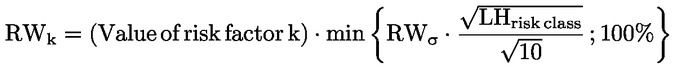In force

Version from: 01/01/2023
Amendments (1)
Search within this legal act

### Article 325ax - Vega and curvature risk weights

Article 325ax

Vega and curvature risk weights

1.
Vega risk factors shall use the delta buckets referred to in Subsection 1.
2.
The risk weight for a given vega risk factor k shall be determined as a share of the current value of that risk factor k which represents the implied volatility of an underlying, as described in Section 3.
3.

The share referred to in paragraph 2 shall be made dependent on the presumed liquidity of each type of risk factor in accordance with the following formula:where:

RWk = the risk weight for a given vega risk factor k;
RWσ shall be set at 55 %; and
LHrisk class is the regulatory liquidity horizon to be prescribed in the determination of each vega risk factor k. LHrisk class is determined in accordance with the following table:

Table 11

 Risk class Risk weights GIRR 60 100 % CSR non-securitisations 120 100 % CSR securitisations (ACTP) 120 100 % CSR securitisations (non-ACTP) 120 100 % Equity (large cap and indices) 20 77,78 % Equity (small cap and other sector) 60 100 % Commodity 120 100 % Foreign exchange 40 100 %
4.
Buckets used in the context of delta risk in Subsection 1 shall be used in the curvature risk context unless specified otherwise in this Chapter.
5.
For foreign exchange and equity curvature risk factors, the curvature risk weights shall be relative shifts equal to the delta risk weights referred to in Subsection 1.
6.
For general interest rate, credit spread and commodity curvature risk factors, the curvature risk weight shall be the parallel shift of all the vertices for each curve on the basis of the highest prescribed delta risk weight referred to in Subsection 1 for the relevant risk class.